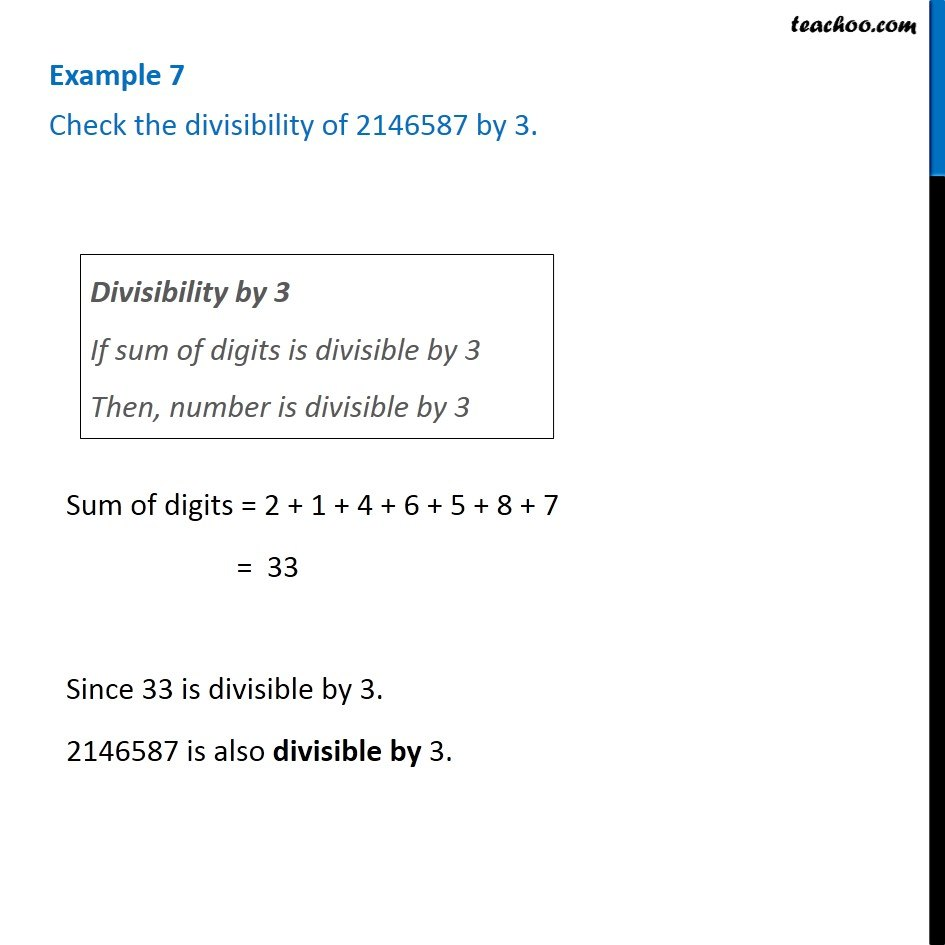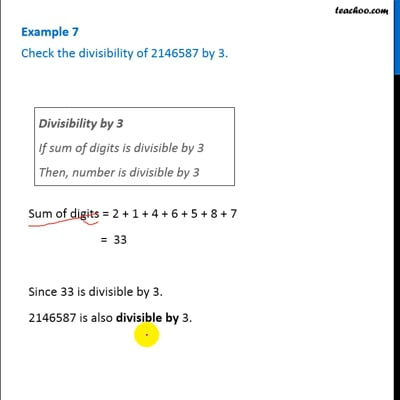Examples

Chapter 16 Class 8 Playing with Numbers
Serial order wiseThis video is only available for Teachoo black users

### Transcript

Example 7 Check the divisibility of 2146587 by 3. Divisibility by 3 If sum of digits is divisible by 3 Then, number is divisible by 3 Sum of digits = 2 + 1 + 4 + 6 + 5 + 8 + 7 = 33 Since 33 is divisible by 3. 2146587 is also divisible by 3.